#### Electron Dynamics, Physics tutorial

Introduction:

The Fermi surfaces (FS) theory lets us to visualize the relative fullness or occupation of the allowed empty lattice bands geometrically in the k-space and therefore assists in the theoretical determination of the electronic properties of the solid- metal, semiconductor or insulator. However, the main aim of the FS construction is to recognize about the details of the motion of the itinerant electron in (3-D) three-dimension.

Definition:

Electron dynamics is employing classical equations of motion in a classical manner to illustrate the electronic structure quantum-mechanically, that is, standing waves which distribute electrons to different areas of the bands.

Electro dynamics:

Given the functions En(k) the semi-classical model relates with each electron a position, a wave vector and a band index  'n'. In the presence of applied fields the position, the wave vector, and the index are taken to evolve according to the given rules:

1) The band index is a constant of the motion. The semi-classical model avoids the chance of interband transitions. This means that in this model it supposed that the applied electric field is small.

2) The time advancement of the position and the wave-vector of an electron having band index 'n' are determined via the equations of motion: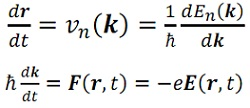Now, we have to prove the above equation. This is similar to the Newton's second law if we suppose that the electron momentum is equivalent to (h/2π) k. The major fact is that, the electrons belong to specific bands which make their movement in the applied electric field dissimilar from that of free electrons. For illustration, if the applied electric field is independent of the time, according to the above equation, the wave vector of the electron rises uniformly with respect to time.

k(t) = k(0) - eEt/(h/2π)

As energy and velocity are periodic in the reciprocal lattice, the velocity and the energy will be oscillatory. This is in striking dissimilarity to the free electron case, where 'v' is proportional to the 'k' and grows linearly in time.

The 'k' dependence (and, to in a scale factor, the 't' dependence) of the velocity is described in the figure shown below. Here both E(k) and v(k) are plotted in (1-D) one dimension. However, the velocity is linear in 'k' close to the band minimum, it reaches an utmost as the zone boundary is approached, and then drops back down, going to zero at the zone edge. In the area between the maximum of 'v' and the zone edge the velocity actually reduces with increasing 'k', in such a way that the acceleration of the electron is opposite to the externally applied electric force!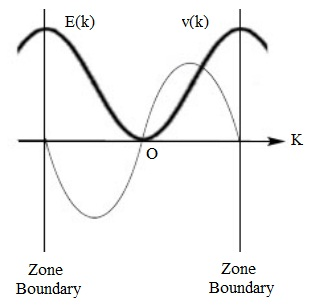Fig: E(k) and v(k) versus k in one dimension Effective mass

This extraordinary behavior is an effect of the additional force applied by the periodic potential, that is comprised in the functional form of E(k). As an electron approaches a Bragg plane, the external electric field moves it in the opposite direction because of the reason of Bragg-reflection.

Effective mass:

Whenever illustrating electron dynamics in solids it is often suitable to introduce the theory of effective mass. If we differentiate the very first equation of the chapter with respect to time we get: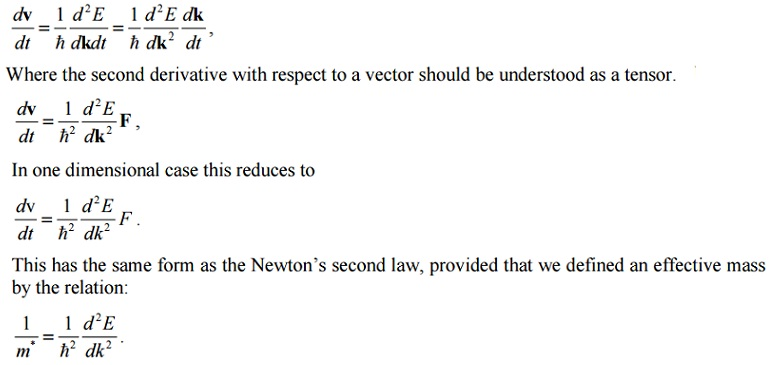The mass 'm*' is inversely proportional to the curvature of the band; here the curvature is large -that is, d2E/dk2 is large - the mass is small; a small curvature means a large mass (as shown in figure given below).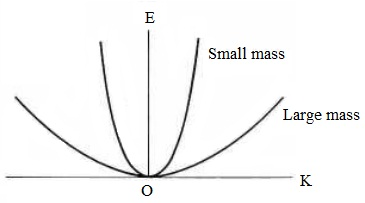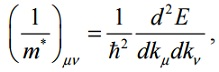Here kµ and kν are the Cartesian coordinates.

The effective mass can be different based on the directions on the crystal.

Current density:

The current density in a free electron model was stated as j = - env, here 'n' is the number of valence electrons per unit volume and 'v' is the velocity of electrons. This expression can be generalized to the case of Bloch electrons. In this condition, the velocity basically depends on the wave vector and we require summing up over 'k' vectors for which there are occupied states available:

j = -e/V k,occupiedΣ v(k)

Here the sum is carried out within the extended zone scheme and 'V' is the volume of the solid. This is often convenient to substitute the summation by the integration. As the volume of k-space per allowed 'k' value is ?k = 8π3/V, we can represent the sum over k as:

Σk = V/8π3 ∫dk

Taking into consideration the spin degeneracy we obtain for the current density:

j = - e ∫occupied dk/4π3 v(k)

By employing this expression we now illustrate that completely filled bands don't contribute to the current. For the filled bands the above equation must be substitute by:

j = -e ∫zone (dk/4π3) {dE(k)/dk}

This vanishes as an effect of the theorem which the integral over any primitive cell of the gradient of a periodic function should vanish.

Hole:

One of the most striking accomplishments of the semi-classical model is its description for the phenomena that free electron theory can account for only if the carriers encompass a positive charge.

We now illustrate the main concept of a hole.

The contribution of the entire electrons in a given band to the current density is represented by the equation j = - e ∫occupied dk/4π3 v(k), where the integral is over all occupied levels in the band. By exploiting the fact that a completely filled band carries no current,

0 = ∫zone (dk/4π3) v(k) = ∫occupied (dk/4π3) v(k) + ∫unoccupied (dk/4π3) v(k)

We can equally well represent the equation j = - e ∫occupied dk/4π3 v(k), in the form:

j = +e ∫unoccupied (dk/4π3) v(k)

Therefore the current generated by electrons occupying a particular set of levels in a band is exactly the same as the current that would be generated if the specified levels were unoccupied and all other levels in the band were occupied however with particles of charge +e (that is, opposite to the electronic charge).

Therefore, even although the only charge carriers are electrons, we might, whenever it is convenient, consider the current to be taken entirely via fictitious particles of positive charge which fill all such levels in the band that are unoccupied through electrons. The fictitious particles are termed as holes.

It should be emphasized that pictures can't be mixed in a given band. If one wishes to regard electrons as carrying the current, then the unoccupied levels make no contribution; whenever one wishes to regard the holes as carrying the current, then the electrons make no contribution. One might, though, regard some bands by employing the electron picture and other bands by employing the hole picture, as suits one's ease.

Generally it is well-situated to consider transport of the holes for the bands that are almost occupied, so that just a few electrons are missing. This occurs in semiconductors in which a few electrons are excited from the valence to the conduction bands. Identical to electrons we can propose the effective mass for the holes. It consists of a negative sign.

Tutorsglobe: A way to secure high grade in your curriculum (Online Tutoring)

Expand your confidence, grow study skills and improve your grades.

Since 2009, Tutorsglobe has proactively helped millions of students to get better grades in school, college or university and score well in competitive tests with live, one-on-one online tutoring.

Using an advanced developed tutoring system providing little or no wait time, the students are connected on-demand with a tutor at www.tutorsglobe.com. Students work one-on-one, in real-time with a tutor, communicating and studying using a virtual whiteboard technology.  Scientific and mathematical notation, symbols, geometric figures, graphing and freehand drawing can be rendered quickly and easily in the advanced whiteboard.

Free to know our price and packages for online physics tutoring. Chat with us or submit request at info@tutorsglobe.com